# Der Lauf der Dinge

Here is a great 30 minute “art” film by Peter Fischli and David Weiss. If you ever enjoyed Mousetrap as a kid or Rube Goldberg if you are a bit older then you will love this.

German title Der Lauf der Linge translates to “The Way Things Go” in English. I remember tracking this video down years ago and it was not easy. Thanks to YouTube it is out there for everyone to appreciate.

It may not be as perfected as the now classic 1 million pound Honda Cog ad, but it was 2 guys in a warehouse doing it for the love of doing it. Apparently Fischli and Weiss did get a bit stroppy and accused the Cog ad of plagarism. Sheesh!

Here is the original 2 minute cut of the Honda Cog ad.

Jason.

# Ducks Fractals Variations

After getting the Ducks Fractal algorithm working, I tried some variations to see what other kinds of images can be prduced using similar methods.

Using the info from Algorithmic Worlds the basic code within each pixels iteration loop is as follows;
``` if z.y>0 then z.y:=-z.y; z.x:=z.x+c.x; z.y:=z.y+c.y; z:=Cln(z); ```

The first line converts Z into its complex conjugate if the imaginary component is greater than 0.
The next 2 lines add the constant C to Z.
The last line takes the log of Z.

Those steps result in z = log(Iabs(z)+p) as stated in the Algorithmic World’s blog post linked above and result in images like the following.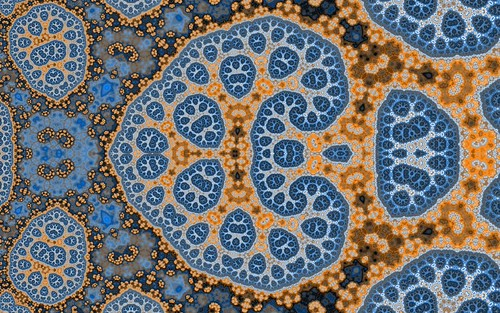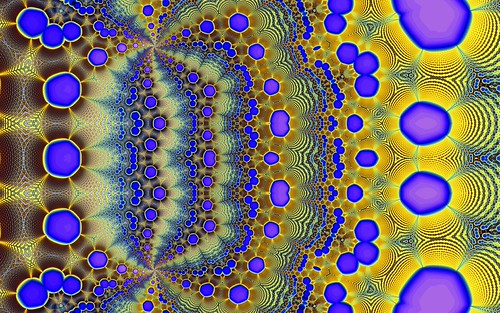Variation 1 uses the following code;
``` if z.y>0 then z.y:=-z.y; z.x:=z.x+c.x; z.y:=z.y+c.y; z:=Cln(CSin(z)); ```

CSin is complex Sin.

Here are a few example images from using this method.Variation 2 uses the following code;
``` if z.y>0 then z.y:=-z.y; z.x:=z.x+c.x; z.y:=z.y+c.y; z:=Cln(Csub(z,CSec(z))); ```

CSub is complex subtraction and CSec is the complex cosecant.

Here are a few example images from using this method.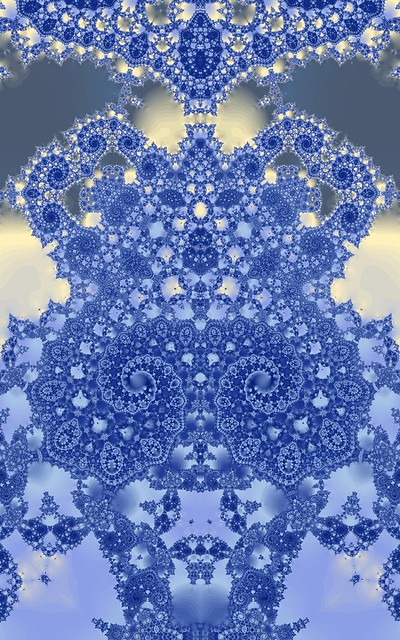Variation 3 uses the following code;
``` if z.y>0 then z.y:=-z.y; z:=CSin(z); z.x:=z.x+c.x; z.y:=z.y+c.y; z:=Cln(z); ```

Here are a few example images from using this method.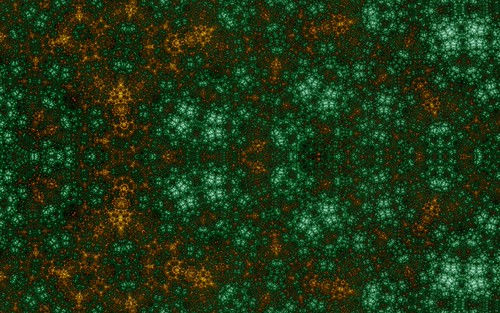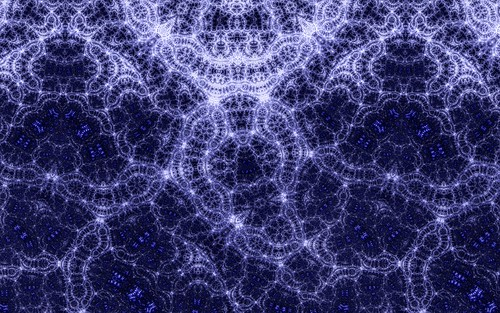Variation 4 uses the following code;
``` if z.y>0 then z.y:=-z.y; z.x:=z.x+c.x; z.y:=z.y+c.y; z:=Cln(CSqr(z)); ```

CSqr is the Z squared.

Here are a few example images from using this method.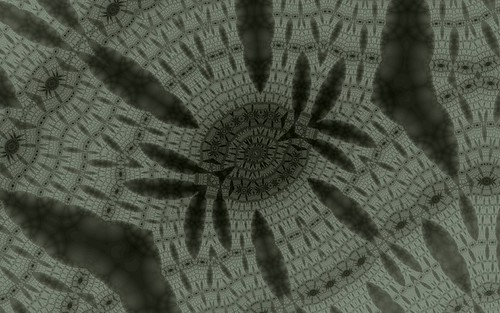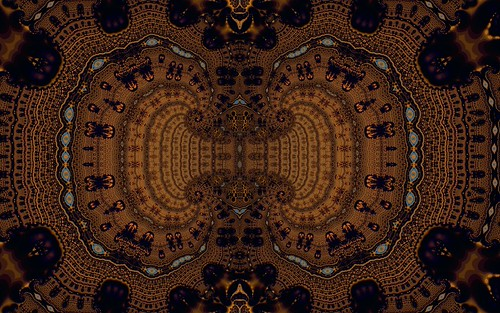All of the above images can (and really need to) be seen in much more detail high resolution images in my flickr gallery.

Ducks Fractals are now included with Visions Of Chaos.

Jason.

# Ducks Fractals

I stumbled across a blog post by Samuel Monnier at Algorithmic Worlds recently that discusses Ducks Fractals. Samuel was also kind enough to share the algorithm and formulas so anyone can have a go at implementing them.

Here are a few of my results using the ducks algorithm.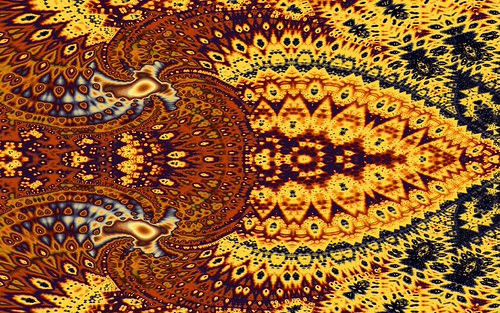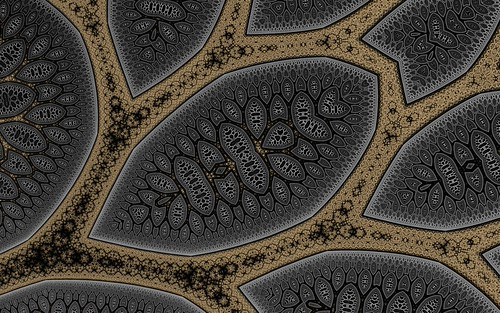To see the above images and more in their original high res versions see my flickr gallery.

Here is a quick test zoom movie into a Ducks Fractal.

Ducks Fractals are now included in Visions Of Chaos.

Jason.